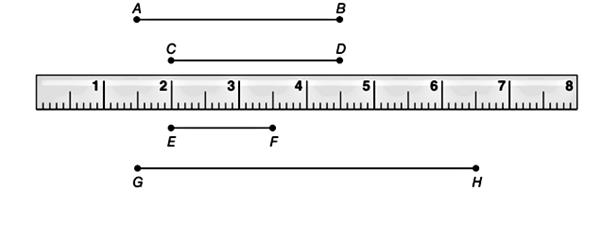Chapter 1.2, Problem 18E### Elementary Geometry for College St...

6th Edition
Daniel C. Alexander + 1 other
ISBN: 9781285195698

#### Solutions

Chapter
Section### Elementary Geometry for College St...

6th Edition
Daniel C. Alexander + 1 other
ISBN: 9781285195698
Textbook Problem
1 views

# Judging from the ruler, estimate the measure of each line segment. a) E F b) G HTo determine

a) To estimate:

The measure of the line segment EF.

Explanation

Calculation:

Given,

Here, the point E of the line segment is at 2 cm and the point F is at 3.5 cm, thus the length of EF¯ is given by

EF=3

To determine

b) To estimate:

The measure of the line segment GH.

### Still sussing out bartleby?

Check out a sample textbook solution.

See a sample solution

#### The Solution to Your Study Problems

Bartleby provides explanations to thousands of textbook problems written by our experts, many with advanced degrees!

Get Started

#### Find more solutions based on key concepts